# Introduction to Heat Exchangers Notes | Study Heat Transfer - Mechanical Engineering

## Mechanical Engineering: Introduction to Heat Exchangers Notes | Study Heat Transfer - Mechanical Engineering

The document Introduction to Heat Exchangers Notes | Study Heat Transfer - Mechanical Engineering is a part of the Mechanical Engineering Course Heat Transfer.
All you need of Mechanical Engineering at this link: Mechanical Engineering

Concentric Pipe Heat Exchange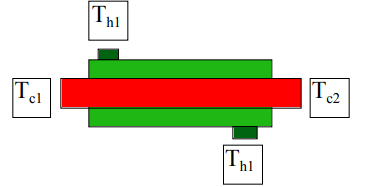Energy Balance on Cold Stream (differential)

dQC = (wCp)c dT= CCdTc

Energy Balance on Hot Stream (differential)

dQH = (wC)H dTH = CHdTH

Overall Energy Balance (differential)

For an adiabatic heat exchanger, the energy lost to the surroundings is zero so what is lost by one stream is gathered by the other.

dQC + dQH = 0

Heat Exchange Equation It follows that the heat exchange from the hot to the cold is expressed in terms of the temperature difference between the two streams

dQ= U (T– TC )dA

The proportionality constant is the “Overall” heat transfer coefficient ( discussion later)

Solution of the Energy Balances

The Energy Balance on the two streams provides a delation for the differential temperature change.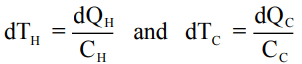However, we should recall that we have an adiabatic heat exchanger so that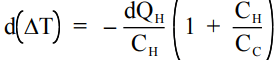Overall Energy balances on each stream

Hot Fluid

QH = CH( TH1 – TH2)

Cold fluid

QC = CC (TC2 – TC1)

Overall Energy balance on the Exchanger

QC + QH = 0

The equation for DT can be modified using the overall energy balances to yield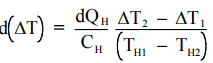The denominator is the energy lost by the hot stream, so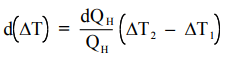.

Application of the relation for energy transfer between the two streams yields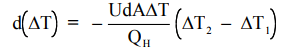Integration of the relation is the basis of a design equation for a heat exchanger.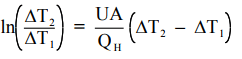Rearrangement of the equation leads to

The Design Equation for a Heat Exchanger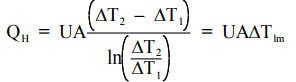Design of a Parallel Tube Heat Exchanger

The Exchanger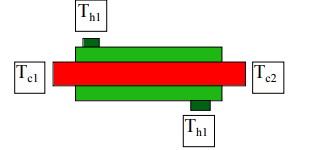The Design Equation for a Heat Exchanger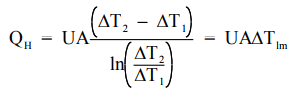Glycerin-water solution with a Pr = 50 (at 70 °C) flows through a set of parallel tubes that are plumbed between common headers. We must heat this liquid from 20 °C to 60°C with a uniform wall temperature of 100 °C. The flow rate, F, is 0.002 m3 /sec (31.6 gal/sec.).

•  How many parallel tubes are required ?
•  How do we select L and D for these tubes ?

Data

The heat capacity, Cp , is 4.2 kJ/kg-°K

The density, r, is 1100 kg/m3

The liquid has a kinematic viscosity, n = 10-3 cm2 /sec.

Step 1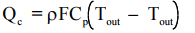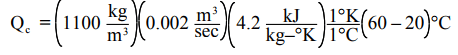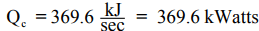Step 2

Calculate the heat transfer coefficient If the flow is laminar, likely since glycerin is quite viscous, and the Re < 2000 the Nusselt number relation for laminar flow can be expressed as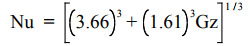The Graetz number is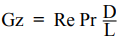If the flow is turbulent (Re > 2000), the Nusselt numberr is given by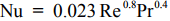We do not know the flow per tube and therefore we do not know the Re. However we don’t need to know that. In Lecture 27 we observed for Heat Transfer in a Tube that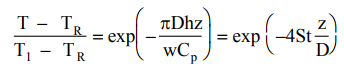The definition of the Stanton Number is :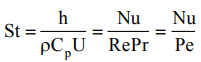Given a Re and Pr, we can calculate the Nu and the Stanton Number, the latter prviding us with the temperature at length L from the previous equation. Let’s examine several configurations at L/D = 50, 100, 200. The Excel table below can be used to specify a design chart.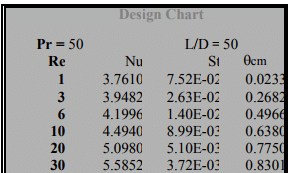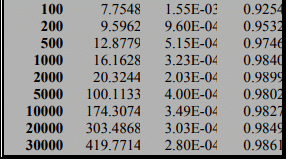To obtain the numbers in the spreadsheet, we used the Nusselt number relation for laminar flow expressed as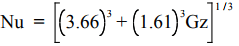and for turbulent flow as

Nu = 0.023Re0.8Pr0.4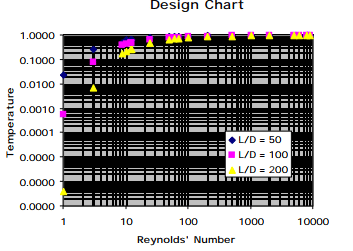Step 3

Calculate the Area required Base case D = 2 cm. and L = 100 D = 2 meters For this case we observe that from the calculations for θcm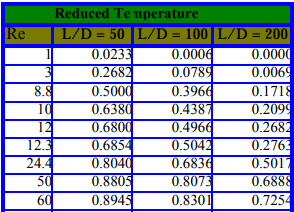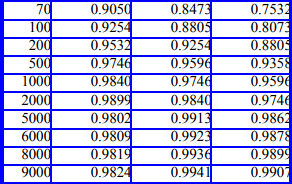We can observe that the flow rate per tube is given by

Fnt = F/ nt

so that the Reynolds’ number is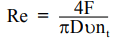As a consequence we can observe that the total length of tubing is not dependent on D alone but on othere considerations that might set a condition for Re, e.g. a pressure drop limitation. Wv find that for this base case, we find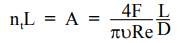We find that θcm = 0.5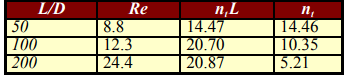Does it make sense?

Maximum Cooling Capacity of an Exchanger of Fixed Area

Water is available for use as a coolant for an oil stream in a double-pipe heat exchanger.

The flow rate of the water is 500 lbm/hr.

The heat exchanger has an area of 15 ft2 .

The oil heat capacity, Cpo, is 0.5 BTU/lb-°F

The overall heat transfer coefficient, U, is 50 BTU/hr-ft2 -°F

The initial temperature of the water, Tw0, is 100°F

The maximum temperature of the water is 210°F

The initial temperature of the oil, Tw0, is 250°F

The minimum temperature of the oil, Tw0, is 140°F

Estimate the maximum flow rate of oil that may be cooled assuming a fixed flow rate of water at 500 lbm/hr There are two possible modes of operation Co-current flow Counter-current flow Let us look at both cases

Co-current flow

Constraints

Tw < 210 ; Tw < To ; To ≥ 140

Energy balances Oil

Qo = FoCpo (To1 – To2 )= Fo (0.5 ) (250 – To2)

Water

Qw = FwCpw (Tw1 – Tw2)

FoCp0 (To1 – To2 )= 500(1.0)(210 – 100) = 55,000 BTU / hr

Recall the Design equation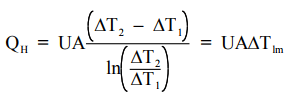Now the ΔTlm is given by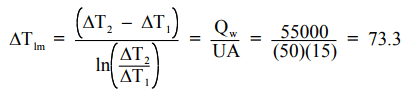Using the temperatures, we obtain T0max = 238.5 °F and from the heat balance for oil, we obtain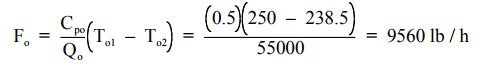Counter-current Flow

Constraints

Tw < 210 ; Tw < To ; To ≥ 140

Energy balances Oil

Qo = FoCpo (To1 – To2 )= Fo (0.5 ) (250 – To2)

Water

Qw = FwCpw (Tw1 – Tw2)

FoCp0 (To1 – To2 )= 500(1.0)(210 – 100) = 55,000 BTU / hr

FoCp0 (To1 – To2 )= 500(1.0)(210 – 100) = 55,000 BTU / hr

Recall the Design equationNow the ΔTlm is given byUsing the temperatures, we obtain T0max = 221 °F and from the heat balance for oil, we obtain the oil flow rate as 3800 lbm/hr.

The document Introduction to Heat Exchangers Notes | Study Heat Transfer - Mechanical Engineering is a part of the Mechanical Engineering Course Heat Transfer.
All you need of Mechanical Engineering at this link: Mechanical EngineeringUse Code STAYHOME200 and get INR 200 additional OFF

## Heat Transfer

58 videos|70 docs|85 tests

Track your progress, build streaks, highlight & save important lessons and more!

,

,

,

,

,

,

,

,

,

,

,

,

,

,

,

,

,

,

,

,

,

;